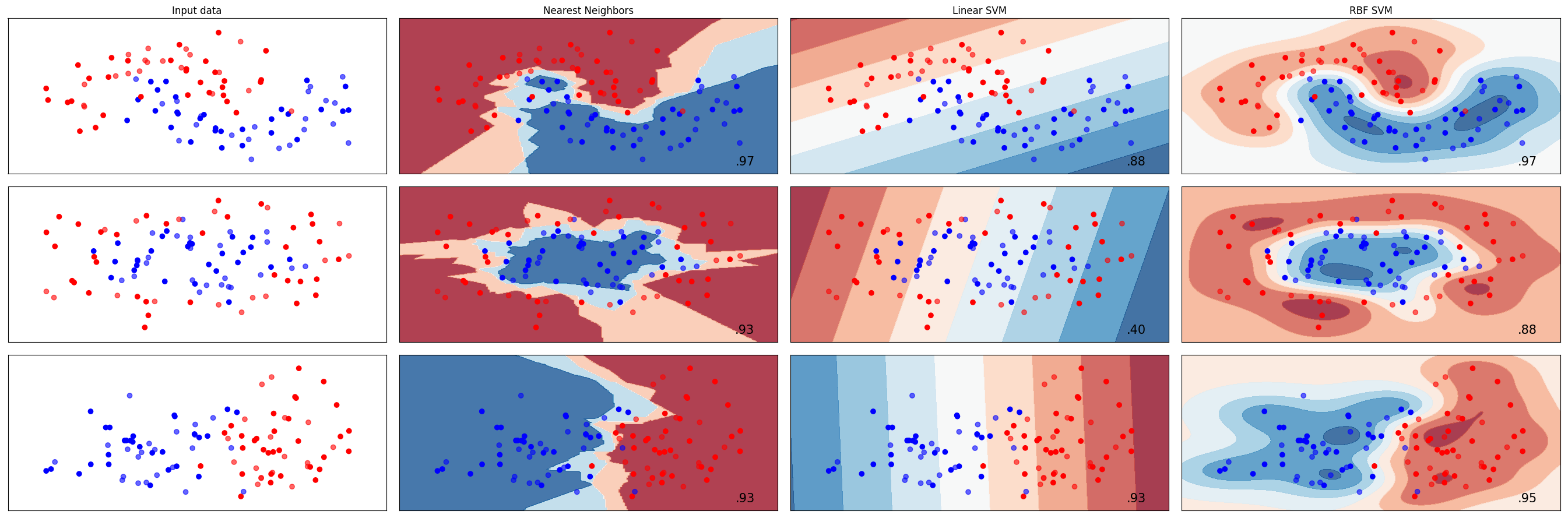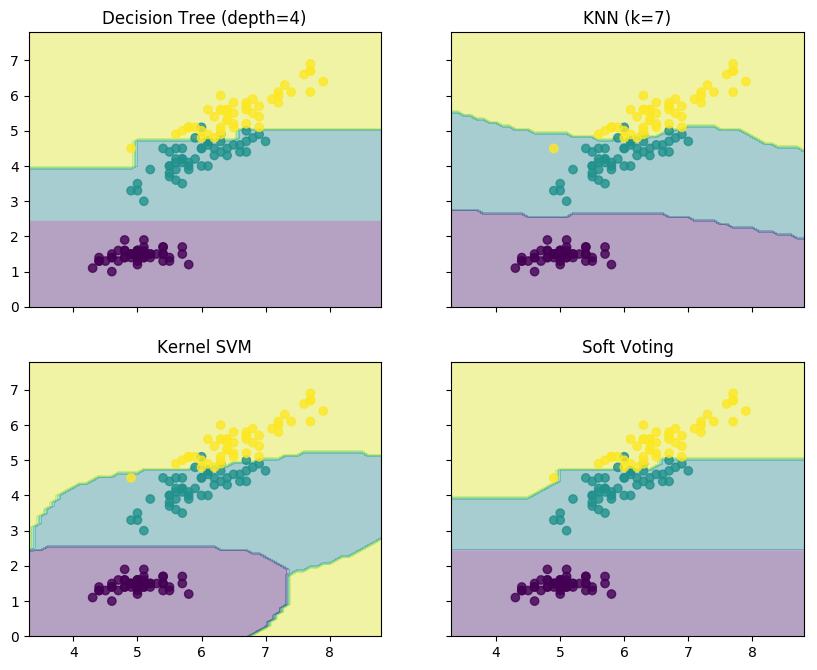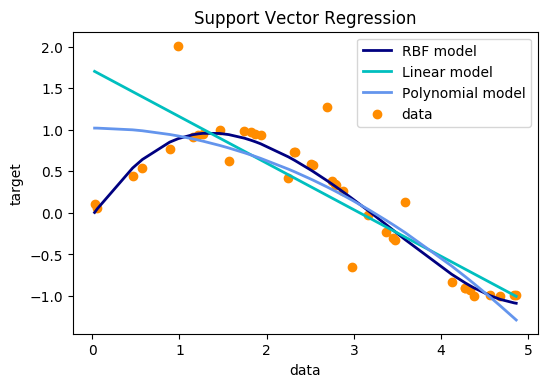# 机器学习系列4：进阶算法-SVMSVM学习方法包含构建由简至繁的模型：线性可分向量机、线性支持向量机及非线性支持向量机，简单模型是复杂模型的特殊情况。当训练数据可分时，通过硬间隔最大化学习一个线性分类器，即线性可分支持向量机；当数据近似线性可分时，通过软间隔最大化学习一个线性分类器，即线性支持向量机；当数据线性不可分时，通过核技巧及软间隔最大化学习非线性支持向量机。

### 线性可分## 1 线性可分支持向量机：### 1.1 函数间隔和几何间隔

在超平面确定的情况下，可以相对地表示点距离超平面的远近。对于两类分类问题，如果，则的类别被判定为1；否则判定为-1。所以如果，则认为的分类结果是正确的，否则是错误的。且的值越大，分类结果的确信度越大。反之亦然。所以样本点与超平面之间的函数间隔定义为SVM训练分类器的方法是寻找到超平面，使正负样本在超平面的两侧，且样本到超平面的几何间隔最大。所以SVM可以表述为求解下列优化问题### 1.2 间隔最大化

间隔最大化的直观解释是：对训练集找到几何间隔最大的超平面意味着以充分大的确信度对训练数据进行分析，在满足将不同类别数据点分开的情况下，而且对最难分的数据点（离超平面最近的点）也有足够大的确信度将他们分开。面最近的点）也有足够大的确信度将他们分开。

### 1.3 对偶问题

为了求解线性可分支持向量机的最优化问题，可以将它作为原始最优化问题，应用拉格朗日对偶性，通过求解对偶问题得到原始问题的最优解，这就是线性可分支持向量机的对偶算法。这样做的优点，一是对偶问题往往更加容易求解：而是自然引入核函数，进而推广到非线性分类问题。### 1.4 线性可分支持向量机的步骤

1、构造带约束的优化问题：2、计算原始问题的最优解：3、求分离超平面：，得到分类决策函数：## 2 线性支持向量机：

线性可分问题的支持向量机学习方法，对线性不可分训练数据不使用。我们需要修改硬间隔最大化，并使其成为软间隔最大化。线性支持向量机是针对线性不可分的数据集的，这样的数据集可以通过近似可分的方法实现分类。对于这样的数据集，类似线性可分支持向量机，通过求解对应的凸二次规划问题，也同样求得分离超平面，以及相应的分类决策函数### 2.1 线性支持向量机的原理### 2.2 线性支持向量机的求解过程

1、设置惩罚参数，并求解对偶问题：。假设求得最优解；

2、计算原始问题的最优解：，选择选择中满足中满足的分量，计算：3、求分离超平面和分类决策函数。分离超平面为：，分类决策函数为：## 3 非线性支持向量机：

前面介绍了支持向量机的基本概念，线性可分支持向量机的原理以及线性支持向量机的原理，线性可分支持向量机是线性支持向量机的基础。对于线性支持向量机，选择一个合适的惩罚参数，并构造凸二次规划问题。求得原始问题的对偶问题的最优解，由此可求出原始问题的最优解：### 3.1 非线性问题的处理方法

在处理非线性问题时，可以通过将分线性问题转化成线性问题，并通过已经构建的线性支持向量机来处理。如下图所示：(非线性转成线性问题)

### 3.2 核函数的定义及常见形式是输入空间(欧式空间的子集或离散集合)，又设为特征空间(希尔伯特空间)，如果存在一个从的映射，使得对所有，函数满足条件。则称为核函数，为映射函数。### 3.3 算法

1、 选取适当的核函数和适当的参数，构造原始问题的对偶问题：， 求得对应的最优解2、 选择的一个满足的分量，求:：3、构造决策函数In :
```import plotly.plotly as py              # for sending things to plotly
import plotly.tools as tls              # for mpl, config, etc.
from plotly.graph_objs import *         # __all__ is safely defined
import plotly.graph_objs as go
import pandas as pd
from pandas import Series,DataFrame
import numpy as np
import datetime

py.sign_in('DemoAccount','2qdyfjyr7o')
```

## 核函数

In :
```def get_y1(x):
return (25-x**2)**0.5
def get_y2(x):
return (100-x**2)**0.5
```
In :
```symbol = np.random.random(100)
```
In :
```symbol[symbol>0.5] = 1
symbol[symbol<=0.5] = -1
# 添加一点小噪音也没多大的意思
x1 = np.linspace(-5, 5, 100)
x2 = np.linspace(-10, 10, 100)
y1 = []
y2 = []
for x in x1:
y1.append(get_y1(x))
for x in x2:
y2.append(get_y2(x))
y1 = np.array(y1)*symbol
y2 = np.array(y2)*symbol
```
In [ ]:
```trace0 = go.Scatter(
x = x1,
y = y1,
name = '类别1',
mode='markers',
marker=dict(
size=12,
line=dict(
color='rgba(217, 217, 217, 0.14)',
width=0.5
),
opacity=0.8
)
)

trace1 = go.Scatter(
x = x2,
y = y2,
name = '类别2',
mode='markers',
marker=dict(
color='rgb(127, 127, 127)',
size=12,
symbol='circle',
line=dict(
color='rgb(204, 204, 204)',
width=1
),
opacity=0.9
)
)

data = [trace0, trace1]

layout = dict(title = '原数据',
yaxis = dict(zeroline = False),
xaxis = dict(zeroline = False)
)

fig = dict(data=data, layout=layout)
py.iplot(fig, filename='原数据集')
```In :
```# 进行核变换
a1 = x1**2
a2 = y1**2
a3 = y1
b1 = x2**2
b2 = y2**2
b3 = y2
```
In [ ]:
```import plotly.plotly as py
import plotly.graph_objs as go
trace1 = go.Scatter3d(
x=a1,
y=a2,
z=a3,
name = '类别1',
mode='markers',
marker=dict(
size=12,
line=dict(
color='rgba(217, 217, 217, 0.14)',
width=0.5
),
opacity=0.8
)
)

trace2 = go.Scatter3d(
x=b1,
y=b2,
z=b3,
name = '类别2',
mode='markers',
marker=dict(
color='rgb(127, 127, 127)',
size=12,
symbol='circle',
line=dict(
color='rgb(204, 204, 204)',
width=1
),
opacity=0.9
)
)
data = [trace1, trace2]
layout = go.Layout(
margin=dict(
l=0,
r=0,
b=0,
t=0
)
)
fig = go.Figure(data=data, layout=layout)
py.iplot(fig, filename='映射后的数据集')
```SVM正文内容

In [ ]:
```
```## 二分类问题和多分类问题

### SVC参数解释

`class sklearn.svm.SVC(C=1.0, kernel='rbf', degree=3, gamma='auto', coef0=0.0, shrinking=True, probability=False, tol=0.001, cache_size=200, class_weight=None, verbose=False, max_iter=-1, decision_function_shape=None, random_state=None)`

</code>

（1）C: 目标函数的惩罚系数C，用来平衡分类间隔margin和错分样本的，default C = 1.0；
（2）kernel：核函数，参数选择有RBF, Linear, Poly, Sigmoid, 默认的是”RBF”;
（3）degree：if you choose ‘Poly’ in param 2, this is effective, degree决定了多项式的最高次幂；
（4）gamma：核函数的系数(‘Poly’, ‘RBF’ and ‘Sigmoid’), 默认是gamma = 1 / n_features;
（5）coef0：核函数中的常数项，’RBF’ and ‘Poly’有效；
（6）probablity: 可能性估计是否使用(true or false)；
（7）shrinking：是否进行启发式；
（8）tol（default = 1e – 3）: svm结束标准的精度;
（9）cache_size: 制定训练所需要的内存（以MB为单位）；
（10）class_weight: 每个类所占据的权重，不同的类设置不同的惩罚参数C, 缺省的话自适应；
（11）verbose: 跟多线程有关，不大明白啥意思具体；
（12）max_iter: 最大迭代次数，default = 1， if max_iter = -1, no limited;
（13）decision_function_shape ： ‘ovo’ 一对一, ‘ovr’ 多对多 or None 无, default=None
（14）random_state ：用于概率估计的数据重排时的伪随机数生成器的种子。

In :
```import numpy as np
X = np.array([[-1, -1], [-2, -1], [1, 0], [2, 1]])
y = np.array([1, 1, 0, 2])
from sklearn.svm import SVC
clf = SVC()
clf.fit(X, y)
print(clf.predict([[-2, -1]]))
print(clf.predict([[1, 0]]))
print(clf.predict([[2, 1]]))
```
```


```

### 实例1（多分类方法对比）¶

In :
```import numpy as np
import matplotlib.pyplot as plt
from matplotlib.colors import ListedColormap
from sklearn.model_selection import train_test_split
from sklearn.preprocessing import StandardScaler
from sklearn.datasets import make_moons, make_circles, make_classification
from sklearn.neural_network import MLPClassifier
from sklearn.neighbors import KNeighborsClassifier
from sklearn.svm import SVC
from sklearn.gaussian_process import GaussianProcessClassifier
from sklearn.gaussian_process.kernels import RBF
from sklearn.tree import DecisionTreeClassifier
from sklearn.naive_bayes import GaussianNB

h = .02  # step size in the mesh

names = ["Nearest Neighbors", "Linear SVM", "RBF SVM"]

classifiers = [
KNeighborsClassifier(3),
SVC(kernel="linear", C=0.025),
SVC(gamma=2, C=1)]

X, y = make_classification(n_features=2, n_redundant=0, n_informative=2,
random_state=1, n_clusters_per_class=1)
rng = np.random.RandomState(2)
X += 2 * rng.uniform(size=X.shape)
linearly_separable = (X, y)

datasets = [make_moons(noise=0.3, random_state=0),
make_circles(noise=0.2, factor=0.5, random_state=1),
linearly_separable
]

figure = plt.figure(figsize=(27, 9))
i = 1
# iterate over datasets
for ds_cnt, ds in enumerate(datasets):
# preprocess dataset, split into training and test part
X, y = ds
X = StandardScaler().fit_transform(X)
X_train, X_test, y_train, y_test = \
train_test_split(X, y, test_size=.4, random_state=42)

x_min, x_max = X[:, 0].min() - .5, X[:, 0].max() + .5
y_min, y_max = X[:, 1].min() - .5, X[:, 1].max() + .5
xx, yy = np.meshgrid(np.arange(x_min, x_max, h),
np.arange(y_min, y_max, h))

# just plot the dataset first
cm = plt.cm.RdBu
cm_bright = ListedColormap(['#FF0000', '#0000FF'])
ax = plt.subplot(len(datasets), len(classifiers) + 1, i)
if ds_cnt == 0:
ax.set_title("Input data")
# Plot the training points
ax.scatter(X_train[:, 0], X_train[:, 1], c=y_train, cmap=cm_bright)
# and testing points
ax.scatter(X_test[:, 0], X_test[:, 1], c=y_test, cmap=cm_bright, alpha=0.6)
ax.set_xlim(xx.min(), xx.max())
ax.set_ylim(yy.min(), yy.max())
ax.set_xticks(())
ax.set_yticks(())
i += 1

# iterate over classifiers
for name, clf in zip(names, classifiers):
ax = plt.subplot(len(datasets), len(classifiers) + 1, i)
clf.fit(X_train, y_train)
score = clf.score(X_test, y_test)

# Plot the decision boundary. For that, we will assign a color to each
# point in the mesh [x_min, x_max]x[y_min, y_max].
if hasattr(clf, "decision_function"):
Z = clf.decision_function(np.c_[xx.ravel(), yy.ravel()])
else:
Z = clf.predict_proba(np.c_[xx.ravel(), yy.ravel()])[:, 1]

# Put the result into a color plot
Z = Z.reshape(xx.shape)
ax.contourf(xx, yy, Z, cmap=cm, alpha=.8)

# Plot also the training points
ax.scatter(X_train[:, 0], X_train[:, 1], c=y_train, cmap=cm_bright)
# and testing points
ax.scatter(X_test[:, 0], X_test[:, 1], c=y_test, cmap=cm_bright,
alpha=0.6)

ax.set_xlim(xx.min(), xx.max())
ax.set_ylim(yy.min(), yy.max())
ax.set_xticks(())
ax.set_yticks(())
if ds_cnt == 0:
ax.set_title(name)
ax.text(xx.max() - .3, yy.min() + .3, ('%.2f' % score).lstrip('0'),
size=15, horizontalalignment='right')
i += 1

plt.tight_layout()
plt.show()
```## 实例2（多分类）¶

In :
```from itertools import product

import numpy as np
import matplotlib.pyplot as plt

from sklearn import datasets
from sklearn.tree import DecisionTreeClassifier
from sklearn.neighbors import KNeighborsClassifier
from sklearn.svm import SVC
from sklearn.ensemble import VotingClassifier

X = iris.data[:, [0, 2]]
y = iris.target

# Training classifiers
clf1 = DecisionTreeClassifier(max_depth=4)
clf2 = KNeighborsClassifier(n_neighbors=7)
clf3 = SVC(kernel='rbf', probability=True)
eclf = VotingClassifier(estimators=[('dt', clf1), ('knn', clf2),
('svc', clf3)],
voting='soft', weights=[2, 1, 2])

clf1.fit(X, y)
clf2.fit(X, y)
clf3.fit(X, y)
eclf.fit(X, y)

# Plotting decision regions
x_min, x_max = X[:, 0].min() - 1, X[:, 0].max() + 1
y_min, y_max = X[:, 1].min() - 1, X[:, 1].max() + 1
xx, yy = np.meshgrid(np.arange(x_min, x_max, 0.1),
np.arange(y_min, y_max, 0.1))

f, axarr = plt.subplots(2, 2, sharex='col', sharey='row', figsize=(10, 8))

for idx, clf, tt in zip(product([0, 1], [0, 1]),
[clf1, clf2, clf3, eclf],
['Decision Tree (depth=4)', 'KNN (k=7)',
'Kernel SVM', 'Soft Voting']):

Z = clf.predict(np.c_[xx.ravel(), yy.ravel()])
Z = Z.reshape(xx.shape)

axarr[idx, idx].contourf(xx, yy, Z, alpha=0.4)
axarr[idx, idx].scatter(X[:, 0], X[:, 1], c=y, alpha=0.8)
axarr[idx, idx].set_title(tt)

plt.show()
```## 回归问题¶

### SVR参数解释¶

`class sklearn.svm.SVR(kernel='rbf', degree=3, gamma='auto', coef0=0.0, tol=0.001, C=1.0, epsilon=0.1, shrinking=True, cache_size=200, verbose=False, max_iter=-1)`

</code>

（1）C: 目标函数的惩罚系数C，用来平衡分类间隔margin和错分样本的，default C = 1.0；
（2）epsilon:不敏感函数，不敏感损失函数，对样本点来说，存在着一个不为目标函数提供任何损失值的区域。默认0.1；
（3）kernel：核函数，参数选择有RBF, Linear, Poly, Sigmoid, 默认的是”RBF”;
（4）degree：if you choose ‘Poly’ in param 2, this is effective, degree决定了多项式的最高次幂；
（5）gamma：核函数的系数(‘Poly’, ‘RBF’ and ‘Sigmoid’), 默认是gamma = 1 / n_features;
（6）coef0：核函数中的常数项，’RBF’ and ‘Poly’有效；
（7）shrinking：是否进行启发式；
（8）tol（default = 1e – 3）: 结束标准的精度;
（9）cache_size: 制定训练所需要的内存（以MB为单位）；
（10）class_weight: 每个类所占据的权重，不同的类设置不同的惩罚参数C, 缺省的话自适应；
（11）verbose: 跟多线程有关；
（12）max_iter: 最大迭代次数，default = 1， if max_iter = -1, no limited。

### 实例3¶

In :
```import numpy as np
from sklearn.svm import SVR
import matplotlib.pyplot as plt
```
In :
```X = np.sort(5 * np.random.rand(40, 1), axis=0)
y = np.sin(X).ravel()
```
In :
```y[::5] += 3 * (0.5 - np.random.rand(8))
```
In :
```svr_rbf = SVR(kernel='rbf', C=1e3, gamma=0.1)
svr_lin = SVR(kernel='linear', C=1e3)
svr_poly = SVR(kernel='poly', C=1e3, degree=2)
y_rbf = svr_rbf.fit(X, y).predict(X)
y_lin = svr_lin.fit(X, y).predict(X)
y_poly = svr_poly.fit(X, y).predict(X)
```
In :
```lw = 2
plt.scatter(X, y, color='darkorange', label='data')
plt.hold('on')
plt.plot(X, y_rbf, color='navy', lw=lw, label='RBF model')
plt.plot(X, y_lin, color='c', lw=lw, label='Linear model')
plt.plot(X, y_poly, color='cornflowerblue', lw=lw, label='Polynomial model')
plt.xlabel('data')
plt.ylabel('target')
plt.title('Support Vector Regression')
plt.legend()
plt.show()
```
```/srv/env/bin/rq-research-kernel:3: MatplotlibDeprecationWarning: pyplot.hold is deprecated.
Future behavior will be consistent with the long-time default:
plot commands add elements without first clearing the
Axes and/or Figure.
# -*- coding: utf-8 -*-
/srv/env/lib64/python3.4/site-packages/matplotlib/__init__.py:917: UserWarning: axes.hold is deprecated. Please remove it from your matplotlibrc and/or style files.
warnings.warn(self.msg_depr_set % key)
/srv/env/lib64/python3.4/site-packages/matplotlib/rcsetup.py:152: UserWarning: axes.hold is deprecated, will be removed in 3.0
warnings.warn("axes.hold is deprecated, will be removed in 3.0")
```In [ ]:

1. 机器学习系列1：算法基础-Logistic回归
2. 机器学习系列2：算法基础-K-Means
3. 机器学习系列3：算法基础-决策树

5. 机器学习系列5：进阶算法-随机森林
6. 数学教学系列6：ARMA模型
7. 数学教学系列7：拉格朗日对偶问题
8. 数学教学系列8：梯度下降法
9. 数学教学系列9：B-S期权定价模型